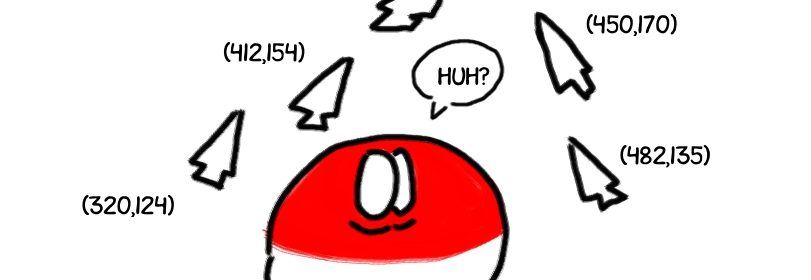# Using mouse for control in MatlabSo, it basically boils down to an old problem: how do I get some user-controlled inputs in Matlab?
Keyboard handling in real-time is basically nonexistant, joystick is alright, but the most easy way to get some input and freedom of controlling things is – to poll the mouse position.

`xy = get( 0, 'PointerLocation' )`

Ok, so everything inside windows in Matlab has a parent-children relationship. Figure 21 contains an axis, axis contains a couple of plots, and so on. But above all those figures there is one root node – the screen itself. You can get the properties of the screen (including the current resolution) as:

```get(0)
```

and among that there is a property that holds ‘PointerLocation’, so the x-y coords of the mouse pointer. To get it:

```mouse = get( 0, 'PointerLocation' )
x = mouse(1)
y = mouse(2)
```

Now for some usage – you can use it in any script that is running in Matlab, for example:

```figure( 21 )
axis
hold on
while(1)
mouse = get( 0, 'PointerLocation' )
plot( mouse(1), mouse(2), '.' )
pause( 0.01 )
end
```

The result? A trace of the mouse path.

Ok, something a little bit more ambitious – a simple cart with a reference position grabbed from mouse:

```function some_silly_name_for_a_file
t = 0:0.01:30;
x = [5;0];

figure(1)
for i=1:length(t)
dx = cart( t(i), x );
x = x + dx*0.01;

plot( x(1), 0, 'o' )
% I assume your screen resolution is 1920
axis( [0 1920 -1 1] )
pause( 0.01 )
end

function dx = cart( t, x )
mouse = get( 0, 'PointerLocation' );
xr = mouse( 1 );
u = 2*(xr-x(1));
dx = [ x(2); -x(2)+u ]
```